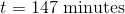# College Chemistry : Galvanic and Electrolytic Cells

## Example Questions

### Example Question #2 : Redox Reactions

Electrolysis of an unknown metal chloride,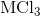, with a current of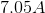for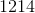seconds deposits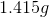of the metal at the cathode. What is the metal?

Scandium

Chromium

Nickel

Titanium

Titanium

Explanation:

Start by writing out the equation that illustrates the plating out of the metal: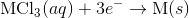Note the stoichiometric ratio for the moles of electrons to the moles of the metal.

Next, recall the following equation: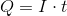, where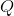is the charge,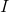is the current, andis the time.

Plug in the given information to find.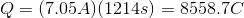Next, recall Faraday's law of electrolysis that equates Faraday's constant and the amount of electrons together to find the charge needed to deposit one mole of a particular substance.

Thus, we can set up the following to take the charge of the electrolysis through to figure out the number of moles unknown metal that was plated out.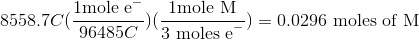Now, since we have the number of grams of the metal deposited, we can find the molar mass of the unknown metal.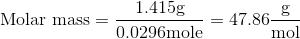The molar mass indicates that the metal must be titanium.

### Example Question #3 : Redox Reactions

Scandium can be plated out of a solution containing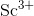according to the following half reaction: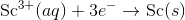How many minutes would it take to plate out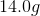of scandium using a current of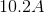?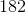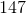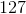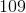Explanation:

Recall the following formula: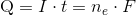, whereis the charge in Coulombs,is the current in Amps,is the time in seconds,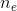is the number of electrons, andis Faraday's constant.

Start by finding how many moles of scandium was plated out.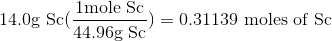Now, use the stoichiometric ratio present in the half reaction to find the number of moles of electrons.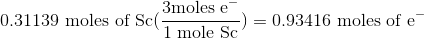Now, use the above formula to solve for the time.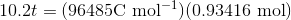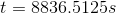Now, convert the seconds to minutes. Remember that your answer should only havesignificant figures.